# Cap percentage values between 0 and 100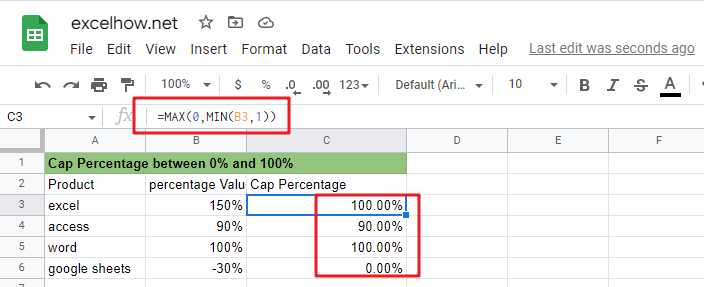If you are a newbie on Excel or google Sheets, you may be able to do this by manually. For tasks with small amounts of data, this method is possible, but for tasks with large amounts of data, this is not a wise method because it will take a lot of your time.

How to quickly cap a set of percentage values from 0% to 100%? You can now read the following section and we will talk about how to do this with formulas from Microsoft Excel or google sheets.

## Limiting percentages by MAX and MIN functions

You can use the MIN function in Google Sheets or Microsoft Excel Spreadsheet in combination with the MAX function to cap a given percentage value between 0% and 100%. Or you can do it with the IF nested functions.

Suppose we have a percentage value of 50%, which is greater than 0 and less than 100%, so it should return that percentage value; if the given percentage value is 120%, it is greater than 100%, so it should return 100%; if the given percentage value is -50%, it is less than 0%, so it should return 0%.

The general formula to achieve this task is as follows.

`=MAX(0,MIN(B3,1))`By applying this formula, you can always cap the percentage value between 0% and 100%.

### Formula explanation

Let’s see how this formula works.

#### =MIN(B3,1)

MIN function can be used to cap a given percentage value greater than 100%, returning 100%.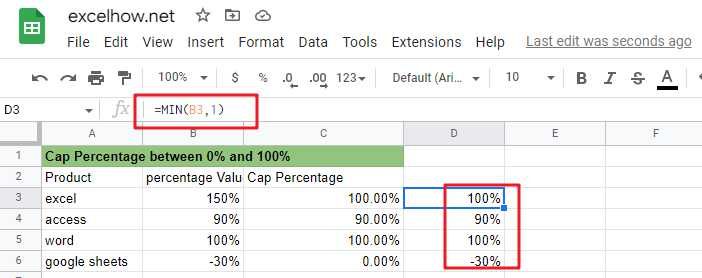#### =MAX(0,B3)

MAX function can be used to limit the given percentage value less than 0, return 0%.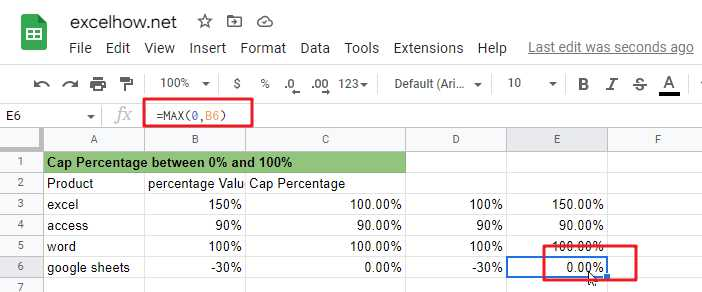## Limiting the percentage value by IF nested functions

You can also limit a given percentage value between 0% and 100% by using the IF nested function. The IF nested function is slightly more complex than the MAX/MIN nested function. There are three kinds of logic.

#### a) limit the value of the percentage does not exceed 100%

by the following IF formula can be used to limit the percentage value greater than 100%, so that the return value of 100%.

`=IF(B3>1,1,B3)`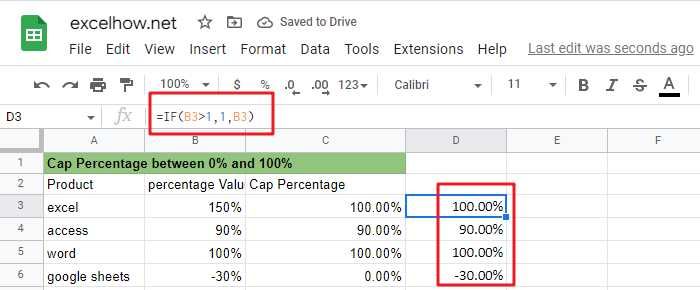#### b) Limit the percentage value not to be less than 0%

The following IF formula can be used to limit the percentage value less than 0, so that its return value is 0%

`=IF(B3<0,0,B3)`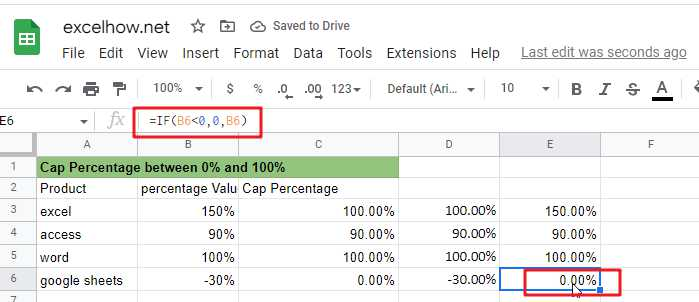#### c) Limit the percentage value that is greater than 0 and less than 100% and returns the original percentage value.

The above three selection logics can be satisfied simultaneously by the following nested IF formulas.

`=IF(B3>1,1,IF(B3<0,0,B3))`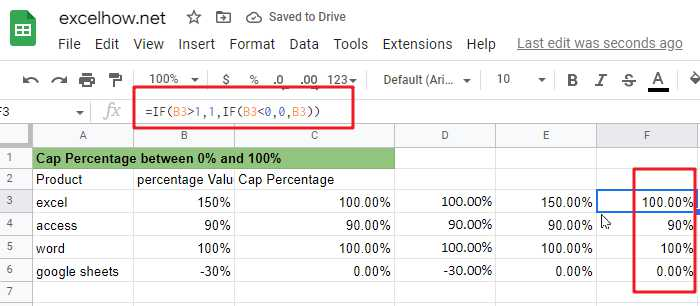From the screenshot above, you can see that the IF nested formula returns the same value as the MAX/MIN formula.